Using DB RTD

# Using DB RTD

## Data Formulas

DB RTD has the following formula format:

```=RTD("db.rtd",,"<Connection string or connection name>","<Database table or view name>"
[,"<Where column>","<Where value>"[,"<Where column>","<Where value>"[,...]]]
,"<Data field>")
```

For example:

```=RTD("db.rtd",,"sqlexpress","dbo.quotes","symbol","AAPL","last")

=RTD("db.rtd",,"sqlexpress","dbo.historical_prices","symbol","AAPL","date",TODAY(),"close")
```

As you can see, DB RTD formulas are similar to the GETPIVOTDATA Excel formula.

In this example, sqlexpress is the name of the connection string. It is a default name for a local Microsoft SQL Server Express database.

You can create named connection strings for your databases in a visual mode using Connection Manager.

Also, you can use connection strings directly. See Connection Strings below.

dbo.quotes and dbo.historical_prices are names of tables or views. You can specify names, including a database like test3.dbo.quotes.

symbol and date are table columns.

close is a data field. DB RTD uses the MAX formula by default. You can specify the aggregate formulas like COUNT(close) or MIN(close).

For the first formula, DB RTD generates and executes the following SQL query:

```SELECT MAX([last]) AS [last] FROM [dbo].[quotes] WHERE [symbol] IN ('AAPL') GROUP BY [symbol]
```

For the second formula, DB RTD generates and executes the following SQL query:

```SELECT MAX([close]) AS [close] FROM [dbo].[historical_prices]
WHERE [symbol] IN ('AAPL') AND [date] IN (<TODAY() value>) GROUP BY [symbol], [date]
```

## Refresh Intervals

You can set the refresh interval for tables using formulas like this:

```=RTD("db.rtd",,"sqlexpress","dbo.quotes","RTD_RefreshInterval",15*60)
```

You can set values in seconds like shown above or as a time value like 0:15:00.

You can call the following function to start refreshing data immediately:

```=RTD("db.rtd",,"rtd_refresh_now")
```

## Tick Fields

Tick fields allow understanding value change directions and can be used with conditional formatting.

Add the :tick suffix to the underlying data field name. For example:

```=RTD("db.rtd",,"sqlexpress","dbo.quotes","symbol","AAPL","last")

=RTD("db.rtd",,"sqlexpress","dbo.quotes","symbol","AAPL","last:tick")
```

## Formula Examples

### Tables with Identity Fields

Tables with identity fields have a single key column like id with auto-incremented value like this: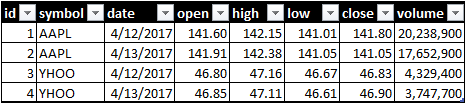Getting values for such tables requires two types of formulas:

1. Getting id values.
2. Getting data values.

To get the last id value for the YHOO symbol, we can use the formula:

```=RTD("db.rtd",,"sqlexpress","test3.dbo.tick_history","symbol","YHOO","id")
```

We can use cell values in regular Excel formulas like this: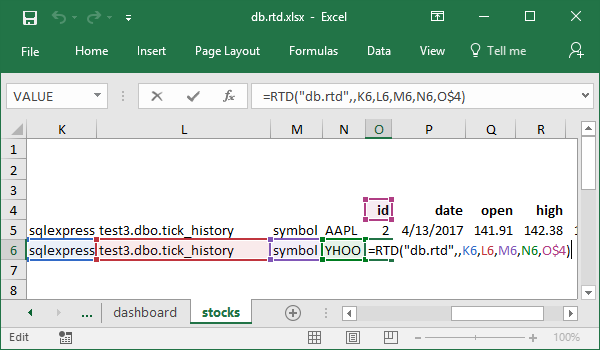In the next steps, we use the id value to get other data. For example:

```=RTD("db.rtd",,"sqlexpress","test3.dbo.tick_history","id",4,"date")
```

The model is below: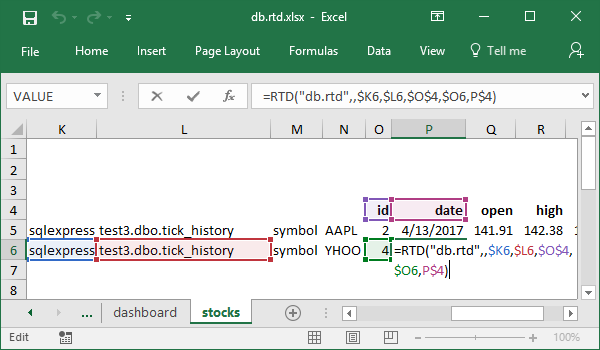### Tables with Multiple Key Fields

The typical scenario is getting historical prices from tables like this: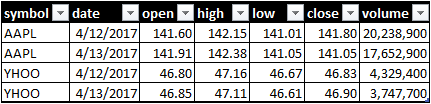To get values for the specific symbol and date, we can use a formula:

```=RTD("db.rtd",,"sqlexpress","test3.dbo.historical_prices","symbol","YHOO","date",TODAY(),"open")
```

To get values for the last trade data, we have to use two types of formulas:

1. the first to get the last date
2. and the second to get data.

For example:

```=RTD("db.rtd",,"sqlexpress","test3.dbo.historical_prices","symbol","YHOO","date")
```

The model is below: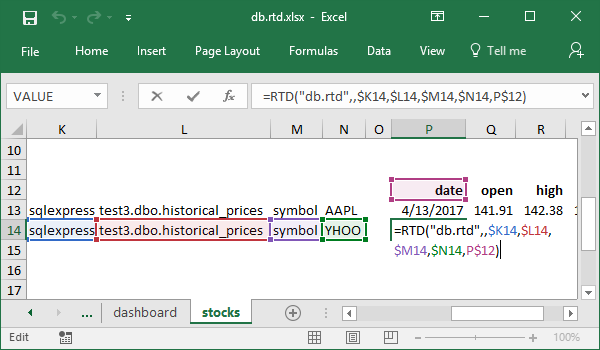The second formula example is

```=RTD("db.rtd",,"sqlexpress","test3.dbo.historical_prices","symbol","YHOO","date","4/13/2017","open")
```

The model is below: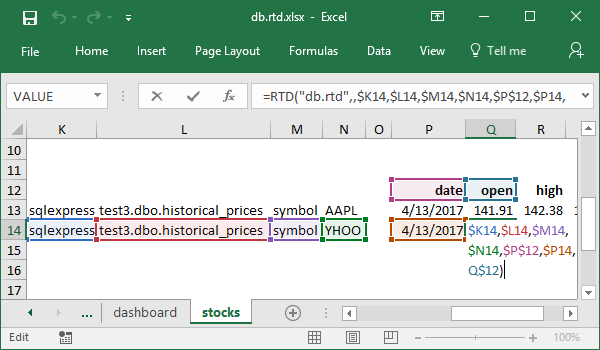### Tables with Single Key Fields

This case is the simplest. The tables have a single primary column like this: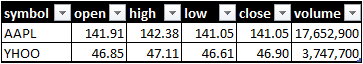You can use a formula like this:

```=RTD("db.rtd",,"sqlexpress","test3.dbo.quotes","symbol","YHOO","open")
```

The model is below: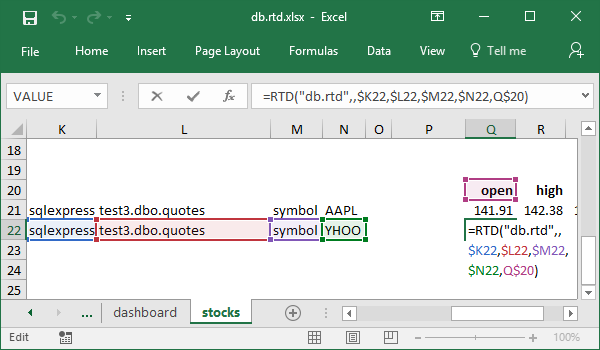## Connection Strings

As mentioned above, you can use connection strings or connection names in the RTD formulas.

Using the connection names is much better as you can create connections in a visual mode and encrypt the connection strings.

However, you can use the connection strings directly in the following forms:

```<.NET provider>;<.NET connection string>

System.Data.OleDb;<OLEDB connection string>

OLEDB;<OLEDB connection string>

<OLEDB connection string>

System.Data.Odbc;<ODBC connection string>

ODBC;<ODBC connection string>

<ODBC connection string>
```

OLEDB is a synonym of System.Data.OleDb and ODBC is a synonym of System.Data.Odbc.

You can omit the OLEDB provider if the OLEDB connection string starts with 'Provider='.

Also, you can omit the ODBC provider if the ODBC connection string starts with 'Driver='.

DB RTD includes .NET providers for Microsoft SQL Server, Oracle Database, MySQL, PostgreSQL, NuoDB, SQL Server Compact, and SQLite.

You have to download and install .NET, OLEDB, or ODBC providers to connect to IBM DB2.

Below are examples of the connection strings.

### Connection Strings for Microsoft SQL Server

```System.Data.SqlClient;Data Source=.\SQLEXPRESS;Initial Catalog=test;Integrated Security=SSPI

System.Data.OleDb;Provider=SQLOLEDB;Data Source=.\SQLEXPRESS;Initial Catalog=test;Integrated Security=SSPI

System.Data.Odbc;Driver=SQL Server;Server=.\SQLEXPRESS;Database=test;Trusted_Connection=Yes

System.Data.Odbc;Driver=SQL Server;Server=.\SQLEXPRESS;Database=test;Pwd=Pa\$\$w0rd!;UID=sa

Driver=ODBC Driver 13 for SQL Server;Server=.\SQLEXPRESS;Database=test;Trusted_Connection=Yes

Driver=ODBC Driver 13 for SQL Server;Server=.\SQLEXPRESS;Database=test;Pwd=Pa\$\$w0rd!;UID=sa
```

### Connection Strings for Oracle Database

```Oracle.DataAccess.Client;Data Source=localhost/test;Password=Pa\$\$w0rd!;User ID=system;

```

### Connection Strings for IBM DB2

```IBM.Data.DB2.10.5.0;Server=localhost;Database=test;UserID=db2admin;Password=Pa\$\$w0rd!

```

You have to install the IBM DB2 .NET provider or ODBC driver on your computer.

Use the "IBM Data Server Client Packages" phrase to find the required IBM DB2 connectivity components.

### Connection Strings for MySQL

```MySql.Data.MySqlClient;Server=localhost;Password=Pa\$\$w0rd!;User ID=root;Database=test

Driver=MySQL ODBC 5.3 ANSI Driver;Server=localhost;Database=mysql;Pwd=Pa\$\$w0rd!;UID=root;OPTION=67108864

Driver=MySQL ODBC 5.3 Unicode Driver;Server=localhost;Database=mysql;Pwd=Pa\$\$w0rd!;UID=root;OPTION=67108864
```

### Connection Strings for PostgreSQL

```Npgsql;Server=localhost;Database=test;User Id=postgres;Password=Pa\$\$word!
```

### Connection Strings for NuoDB

```NuoDb.Data.Client;Server=localhost;User=DBA;Password=goalie;Database=test
```

### Connection Strings for Microsoft SQL Server Compact

```System.Data.SqlServerCe.4.0;Data Source=d:\data\test.sdf;Password=
```

Note that you have to specify the absolute path to the database file.

You can use the following formula to get the path to the file in the active workbook folder:

```="System.Data.SqlServerCe.4.0;Data Source="&amp;LEFT(CELL("filename"),FIND("[",CELL("filename"),1)-1)&amp;"test.sdf;Password="
```

### Connection Strings for SQLite

```System.Data.SQLite;Data Source=d:\data\test.db;Version=3;
```

Note that you have to specify the absolute path to the database file.

You can use the following formula to get the path to the file in the active workbook folder:

```="System.Data.SQLite;Data Source="&amp;LEFT(CELL("filename"),FIND("[",CELL("filename"),1)-1)&amp;"test.db;Version=3;"
```